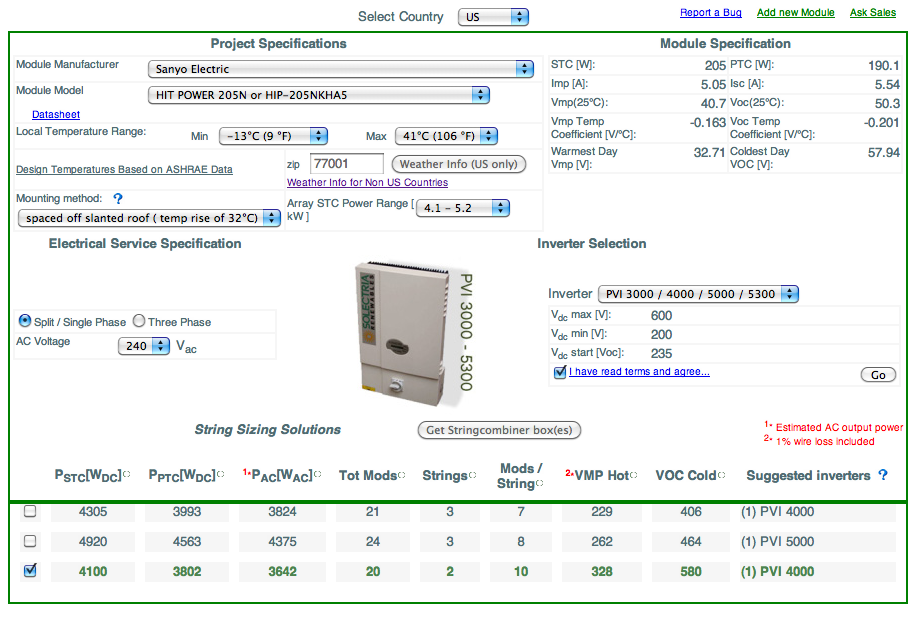This is a the third installment in a three-part series on residential solar PV design. The goal is to provide a solid foundation for new system designers and installers.  This section is dedicated to the basics of inverter sizing, string sizing and conductor sizing.

1. Part 1: How to Design a Solar PV System: The Basic Terms
2. Part 2: How to Design Solar PV – A Walk-Through of Array Sizing and Estimating Power Production
3. If you’re looking to start a solar business, check out our free solar startup guide.

This post is specifically focused on basic technical understanding of solar PV projects. However, more and more we’re getting questions from contractors who need to understand how to finance commercial solar projects.

We also get a lot of questions about NABCEP certification from people looking to design solar projects.

Click here to learn what is NABCEP and whether or not you should need to get the certification. If you’re serious about the solar industry and you want to get the NABCEP Certification, but you need to understand how exactly to apply, you can read more about getting the NABCEP certification here.

The goal of the article is to convey the basic process for sizing an inverter, strings, and the conductors. You may not be an expert at the end of the post, but you’ll have a better understanding of how to do these things.

As always, having specific numbers is the most useful for examples, so we’ll continue with the example from part 2 on sizing an array and estimate power production. The house was located in Houston, TX and the roof, given local shading conditions, has enough room on the roof for 20, 205 watt modules. (Read Part 2 to see how we arrived at this number.)

Here is the specification sheet on the Sanyo HIT 205 module we’ll use for the example.

So, the largest possible size of the array we can fit on the roof at STC is 4,100 watts. We can go lower then this but not higher.

# 1. Inverter Sizing and Selection

Given that we know how many modules can fit on the roof, how do we use this data to size the inverter? The size of the inverter is driven by answering two questions:

1 – What is the capacity of the existing electrical service?

Per NEC 690.64B2 (2008) 705.12 D2 (2011), an existing electrical service is only allowed to backfed up to 120% of the rated capacity.

What does this mean with a typical home?

100 amp service X 20% = 20 amp backfed breaker allowed

16 amps X 240 volts (or 208 volts, depending on the homes location) = 3840 watts. This is the maximum allowed AC power output of the inverter.

There are a few ways of getting around this, by upgrading the service, performing a line-side tap, and it can sometimes be accomplished with subpanels. However, for this example, let’s keep it simple.

If the existing service only  had room for a 20amp breaker, we would not be able to have an inverter that has a rated AC continuous output that would exceed the 16 amp (see example above) or 3840 watts AC.

Per NEC 690.8 A3 the maximum AC output current from an inverter is defined as the manufacturer’s continued rated output current.

Max Current (inverter AC circuits) = continuous current output.

For our example, we’ll assume that the existing electrical service can supply an additional 25 amp back-fed breaker, 20 amps continuous allowed. This limits our choice of inverter to either a PVI 3000 or PVI 4000 inverter based on the electrical service capacity, as the PVI 5000 has a continue output current at 208 VAC of 20.7 amps.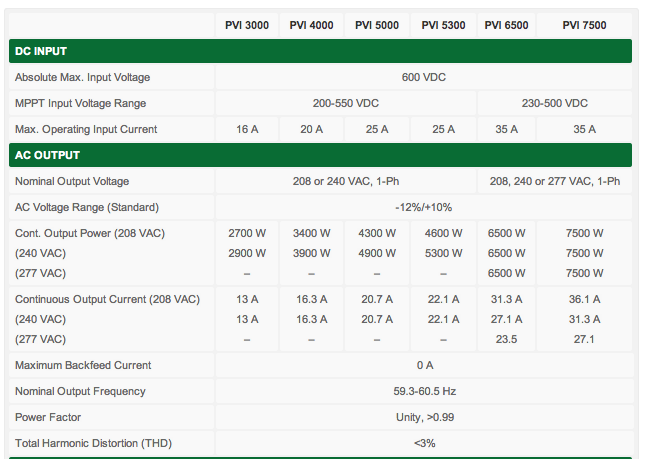Figure 1 – A Sampling of Solectria Residential Inverter Specs

# 2 – How many modules can we fit on the roof?

It’s very critical that you perform proper site visits before design the system, so you know the roof that you’re dealing with.

Performing high quality and efficient site visits is absolutely critical to the success of profitable and well designed solar projects, especially residential projects! You need to be able to capture all of the information you need to 1) quote the system correctly 2) design the project and 3) inform the installation crew what to expect. An efficient site visit process will lead to smooth operations and profitable jobs while complex process can lead to unprofitable jobs and a lot of confusion.

Back to our example. From our example, we know that we can fit 20, 205 watt Sanyo modules on the roof.

Here is the specification sheet for the module: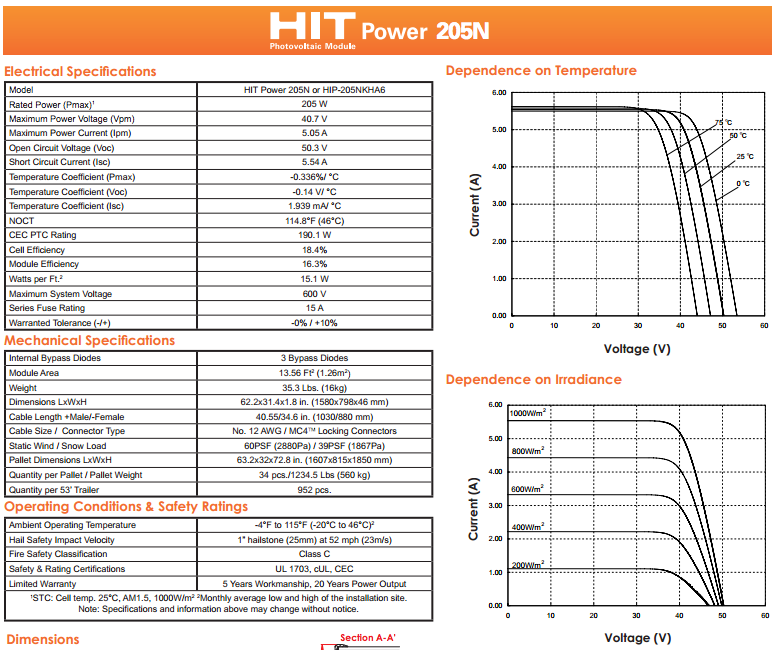Figure 2 – Spec sheet for Sanyo 205 Module

First, we need to guess the size of the inverter. It’s a good rule of thumb to size the inverter, based on the rated AC continuous output, to be 80% smaller then the rated STC output of the array. The reason for this is that there is a lot of inefficiency from the array to the inverter, so if we undersize the inverter, the array is more likely to hitting the upper limit of the input ranges of the inverter and will more likely be operating within the MPPT operating range of the inverter.

For example, for our array size at 4,100 watts DC STC, we’ve guessed that the inverter would have a AC continuous output range of 80% of 4.1kW, or 3,280 watts AC.

You’ll notice that the naming of Solectria inverters (PVI 3000, 4000, 5000) also seem to match this relationship between the DC rated power of an array (the name of the inverter) and the AC continuous output of the inverter (2700W, 3400W, 4300W, respectively)

We will choose the Solectria PVI 4000 for our example from our choices between the PVI 3000 and 4000

3. How do we size the strings?

Right now, we have concluded two things. First, the inverter we’d like to use the PVI 4000 based on the number of modules that can fit on the roof and how their capacity relates to the inverter. Second, we know the number of max modules we can fit on the roof. Now, we must begin string sizing.

String sizing is the number of modules that we will connect in series and parallel before connecting them to the inverter. The size of our strings will determine the voltage and amperage that is inputted into the inverter.

When string sizing, our goals are:

1. Make sure we NEVER supply the inverter with too much voltage, which will kill it –> Maximum string length
2. Make sure that we can ALWAYS supply the inverter with enough voltage to turn on, given the array is receiving full sun –> Minimum string length

What is the maximum voltage allowed for the system? How many modules we can connect in series?

NEC 690.7 specifies that our worst-case voltage, the highest voltages that the DC array can create, must fall within the limits of the inverter.

The exact definition states that: The Voc of each module times the number of modules in a string, correct for lowest expected ambient temperature in the array’s location.

For the PVI 4000, maximum acceptable voltage is 600 VDC.

To calculate the maximum number of modules allowed, we need a few pieces of data

• Voc at STC for the module at 77F/25C = 50.3 volts
• The temperature coefficient for the module. Typically given in volts per degree C or % voltage per degree C. You will find all this data on any module spec sheet = -.14V/C
• The lowest and highest temperatures seen in the specific jurisdiction. Below is the data for Houston from weather.com = 9F or -13CHere are the calculations for the max system string size. The goal in determining the maximum system voltage is to make sure that power production from the array will never kill the inverter.

1. Temperature coefficient. -13C lowest temperature – 25C STC = -38C change from STC
2. -38C  X -.14V/C = 5.32 voltage increase. (negative times a negative is a positive)
3. 50.3 volts + 5.32 = 55.62 is the highest voltage we will ever expect to see from each module, and this is the voltage we will use to determine the maximum number of modules in a string.
4. 600VDC (highest acceptable inverter voltage) / 55.62 = 10.78 modules.
5. We round down to 10 modules, because we cannot go over 600 volts.
6. Maximum system voltage (MSV) = 10 modules X 55.62 = 556 volts

How do we calculate the minimum number of modules in a string?

The goal of calculating the minimum number of modules in a string is to make sure that in the worst case scenario, when the array is extremely hot, the system will still produce enough voltage to turn the inverter ON. Thus, we’re looking to understand the lowest possible voltage the system will create.

Here’s what we need:

1. Vmp of the module. Operating voltage of the module under load = 40.7 volts
2. Temperature coefficient correction factor for the module from STC = -.14V/C
3. The highest temperature recorded for the location you’re installing = 106 degrees
4. The bottom range of acceptable voltage for the MPPT range for the inverter = 200 V DC
5. Ambient air correction factors from the conduit that the electric wire will be in. This can be looked up at NEC Table 310.15 B2C. Based on how far the conduit is off the roof, it will give us the temperature that we need to ADD to the highest temperature to derate module performance. Why? wires heat up more when sitting in conduit rather then outside air.

Here are the calculations.

1. The conduit will be placed 2 inches above the roof. Thus, we must add 40F to the output temperature of 106 to find the temperature we will derate the modules by 40 + 106 = 146. 146F = 63.3C
2. 63.3C – 25C (STC) = 38.3C above STC. Remember, voltage is indirectly related to temperature. Higher temperature equals lower voltage. Thus, the hottest conditions the array will ever see is 38.3C higher then the STC voltage.
3. 38.3C X -.14V/C = 5.36 DECREASE in voltage per module
4.  40.7Vmp – 5.36v = 35.34 Vmp (at 149F) What this means is that is the array is under load (being used) and it’s the hottest that it’s ever been in Houston (106F) and the conduit is 2 inches above the roof, we can expect that each module will be producing 35.34 volts.
5. 200VDC (the minimum volts needed to turn on a PVI 4000) / 35.34Vmp = 5.65 modules. For this we need to ROUND UP (if we go down the inverter won’t turn on) so our conclusion will be 7 modules.

Conclusion on Voltages

With a Sanyo 205 module, we can have between 7 and 10 modules given the voltage ranges of a PVI 4000 inverter.

However, now we need to make a table to figure out how many strings to have and the proper number of strings to produce enough POWER (watts) for the inverter.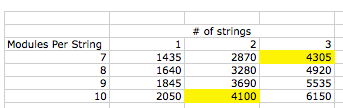We could select either 2 strings of 10 modules or 3 strings of 7 because both will produce enough DC power to power our inverter.

We will select 2 string of 10 modules for two reasons.

• Our roof only has enough space for 20 modules so 21 will not fit on it.
• All else equal, it’s better to have fewer strings and more modules per string because higher voltages = less voltage drop because less amperage will be flowing for the same amount of power.

The conclusion from Solectria’s string inverter tool match our findings done by handString Sizing Tools are Readily Available and Free

All of these calculations are typically done with software or with an inverter manufacturer’s string sizing tool.  Here are three free options:

However, it’s good to understand the theory behind their calculations.

4. How do we size conductors?

After we’ve selected the size of the array and the inverter we need to size the conductors that will be used.

The purpose of conductor sizing is to make sure conductors can hold the amperage that they need to help.  The ampacity rating of a conductor is the current it can safely conduct without overheating. The reason conductors cannot overheat is because the insulation on the outside will melt and the faults will be more likely to occur.

• Current causes heat in conductor due to resistance of the wire
• Bigger wires = lower resistance
• Lower resistance = less heat.
• Too much heat = insulation melting = faults, arcs, death and fire.
• Insulation rating determine ampacity

Most people, including myself, find this extremely confusing at first.  So before we start talking about conductor sizing, let’s take a look at the problem from 30,000 feet to understand logically what is happening:

1. Understand how much the conductor NEEDS TO CARRY. The first thing we need to understand is how many amps need to flow through a section of wire. When looking at solar PV project they come into two main group, solar PV source circuits (those from after the modules and before the inverter) and non-solar PV source circuits (those coming after inverter)
2. Understand how much the conductor CAN CARRY based on it’s rated ampacity AND conditions of use. We’ll talk about how to adjust a wire based on conditions of use and it’s rated capacity.
3. Thus, for every conductor sizing example, we should always be asking ourselves, HOW MUCH does the conductor NEED TO CARRY and HOW MUCH can the conductor carry. In all cases, the conductor MUST be able to carry more then it must carry.

With that in mind, there are four areas that we need to consider when sizing conductors.

1. Standard ampactiy tables based on a) continuous or b) maximum current.
2. Derating wire for conditions of use
3. Fuse or OCPD protector rating
4. Terminal Rating

1. Standard Ampacity Tables based on continuous or maximum current.

Determining ampacity requirements based on continuous or maximum current. Remember this is calculating HOW MUCH a conductor needs to carry.

• Isc = Rated short circuit current which is the maximum current flow when the positive and negative are connected together at STC. Our module has an Isc of 5.54A
• Maximum Current. NEC 690.8A Circuits that are supplied by solar PV modules (anything before the inverter) can deliver output current that is HIGHER than their rated short circuit currents. Rated short circuit is at 1000W/M2 irradiance. Real conditions can see 1250 W/M2. –> Thus Isc X 1.25 = Maximum solar pv source circuit current
• Continuous Current. NEC 690.8B1 and 210.19A1. Continuous loads can only be loaded to 80% of it’s capacity. Solar PV array output AND inverter output are always considered to be continuous since they last for more then 3 hours. Thus, 10amps (max Isc) x 1.25 = 12.5 amp conductor.
To understand which needs to be applied to what circuits, it’s easiest to separate between solar PV circuits (before the inverter) and non-solar PV circuits (after the inverter)
• Solar Generated Circuits = Isc X 1.25 (high current) X 1.25 (continuous load) = Isc X 1.56 = the required conductor ampacity for a solar source circuit
• In our example, the Isc is 5.54 amps and we have 2 strings. Thus, our conductors must be able to carry 5.54 X 1.56 X 2 = 17.28 amps.
• Inverter AC circuits = Rated current X 1.25 (for continuous use) = require conductor amapacity. Note: many inverter manufactures will specify simply the “continuous AC output current”, so you don’t need to perform this calculation. For the PVI4000, that’s 16.3 amps.

2. Derating Wire for Conditions of Use

Now that we understand how to calculate HOW MUCH conductors need to carry, we need to select a conductor that can carry that current in the conditions where it will be used. All else equal, the hotter the surrounding air that conductors are placed in, the less amperage they can safely carry and still meet our ampactiy ratings for safety.

There are three main things to consider:

1. The rated capacity of the wire at testing conditions

2. The effects of temperature where it will be used

3. The effect of conduit fill

1. Standard Conductor Ampacity

The NEC has tables of ampacity for different conductors depending on size and the insulation used. The standard ambient temperature is assumed to be 30C.

• Table 310.16 is for conductors in conduit or earth
• Table 310.17 is for conductors in free air.
Below is a sampling from 310.16

Example: From our example, what is the smallest size wire that can be used from a combiner box that combines 2 strings of Sanyo 205 modules in parallel?

Min Amapacity = Isc X Number of Strings X 1.56 = 5.54 X 2 X 1.56 X = 17.28 amps

This can be satisfied with by a AWG 14 THWN-2 Conductor

Why? We NEED to carry 17.28 amps. AWG14 CAN CARRY 25 amps. 25 > 17.28

If there are more than 3 current carrying conductors in a raceway or cable, the conductor ampacity must be derated for conditions of use per NEC 100, this excludes grounding conductors per NEC 310.15B5

The impact of conduit fill is essentially the same as the increase in temperature, more conductor in a conduit, and where that conduit is, will impact how hot it gets in that conduit and thus how much the conductor can carry.

Table 310.15B2a gives factors for derating. Some value from 310.15 are below.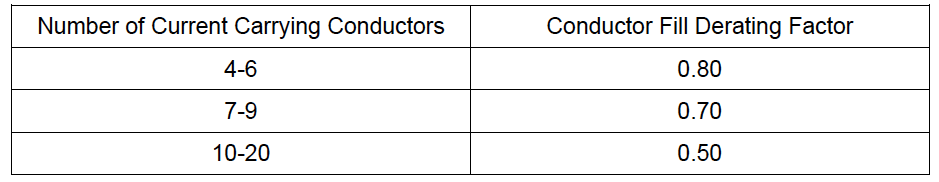From our example, we only have 2 strings ( 4 home runs) from the array. Let’s assume that we have 40 modules (10 modules per string, 4 strings), that we are combining, so we have to combine 2 arrays. 8 source circuits into 4.

What would be the minimum amapcity in that situation?

Number of conductors = 4

Min ampacity needed for two conductors = 17.28

Adjusted ampacity for conduit fill = 17.28 / .80 = 21.6

14AWG still works because it has a maximum capacity of 25 amps.

The conductors are testrd and their ampacity is rated for 30C. Thus, if the conductors will be used in any condition that may be higher then 30C, we need to reduce their ampacity ratings. NEC provides table to perform these calculations in table 310-16 and 310-17.

Below is a sample from 310-16.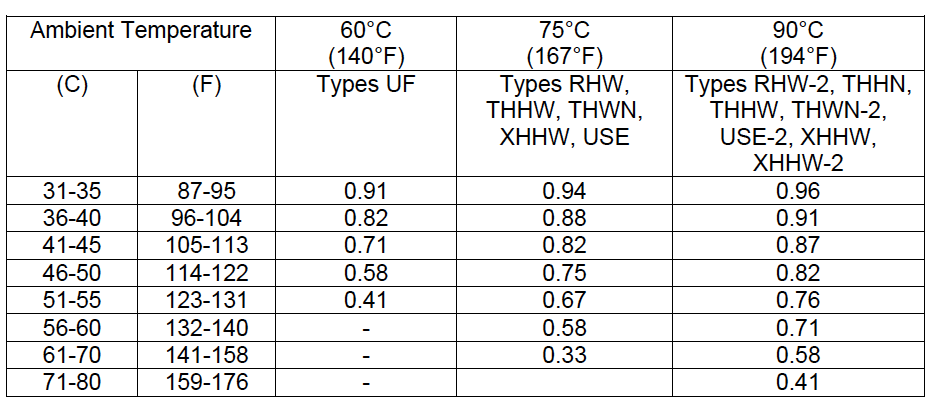Example. Our system will be installed in Houston, TX, the highest temperature is 106F. Can we still use 14AWG to combine our source circuits?

14AWG Standard Amapacity = 25 amps.

Adjusted Ampacity = 25 amps X .87 = 21.75 amps.

This means that given it 106 outside, 14AWG is rated to carry 21.75 amps. We need to carry 17.28 amps. So 14AWG still works.

The temperature derating also needs to be adjusted based on the distance that the conduit is above the roof.

Per NEC 310.15B2C, the below determines the temperature that needs to be ADDED to the highest ambient temperature based on where the conduit is placed.From our above example, if the conduit was being run 1 inch above the roof, we would need to ad 40F to the ambient temperature of 106F to equal 146F.

This means that a 14AWG with a rated capacity of 25 amps will have an ampacity of 25amps X .58 = 14.5 amps.

Let’s walk through a full example to make sure we have all these concepts in order.

What we need to make sure is that corrected amapacity of the conductors (the rated capacity derated for use) is GREATER then the maximum amount of current that will be flowing through the conductor.

We have 2 strings of 10, 205 watt modules

• Minimum Ampacity = 2 parallel strings X Isc X 1.59
• 5.54 X 2 X 1.56 X = 17.28 amps
• 17.28 amps is REQUIRED

We have 2 conductor pairs, 4 home runs running to the DC disconnect and interview. Conduit filled is .80

The highest ambient temperature is 109F/43C

The homeruns will be conduit that is 1/2 inches above the roof. This adds 33C to the 43C ambient temperature equalling. Our temperature derating is now 76C or .41

Thus, the equation is Conductor Ampacity X conduit fill derate X ambient temperature derate.

14AWG is rated for 25 amps.

25 amps X .80 X .41 = 8.2 amps.

Under these conditions 14AWG is only rated to carry 8.2 amps. WE MUST CARRY 17.28 amps.

We would increase our conductor size to #8 AWG

8AWG – 55 amps X .80 X .41 = 18.04 amps.

18.04 conductor ampacity > 17.28 minimum ampacity needed.